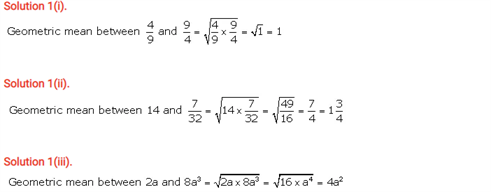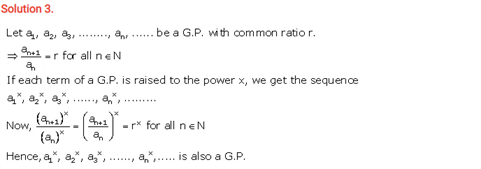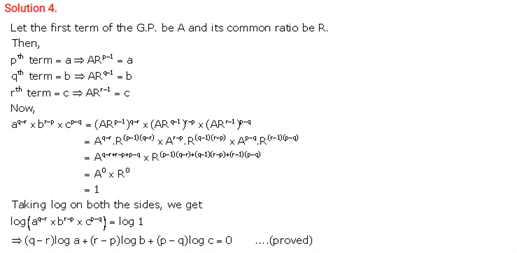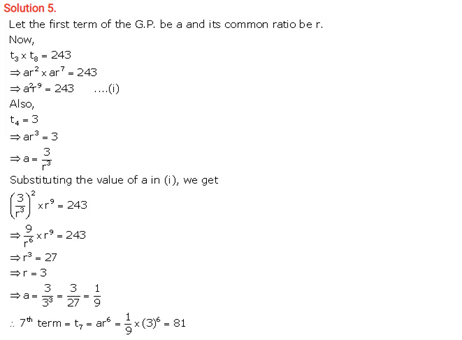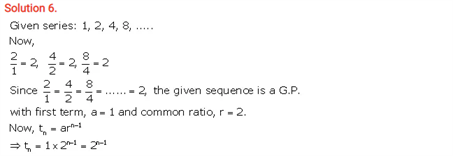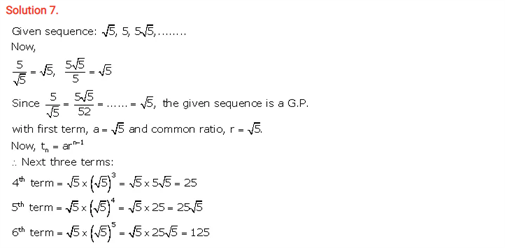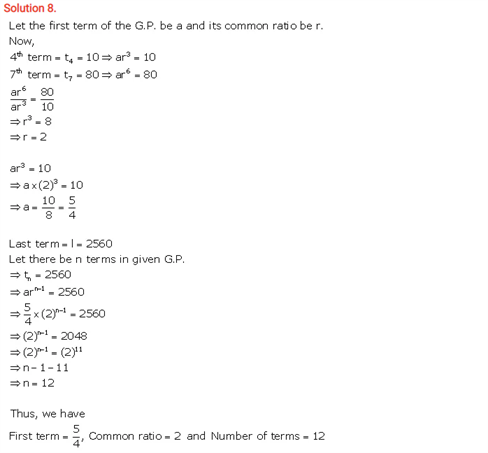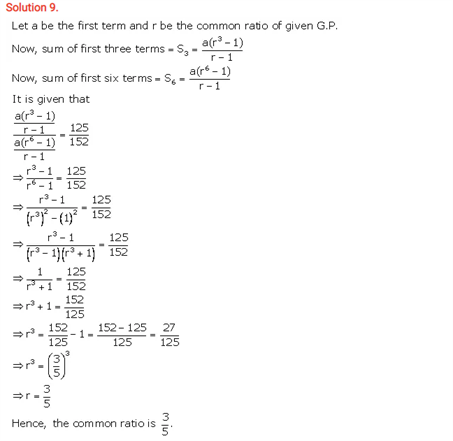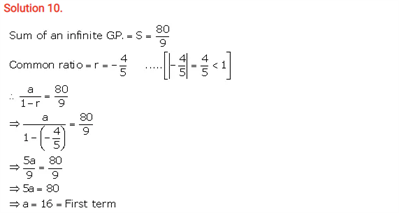# Selina Concise Maths Solution for ICSE Class 10 Chapter 11 Geometric Progression

## Selina Class 10 Maths Solutions for Chapter 11

ICSE Solutions For Class 10 Maths comprises of all the important questions of chapters. A Geometric progression is referred to the sequence of numbers in which after the first element, any element after that can be got by multiplying the preceding element by a constant known as a common ratio. Similarly, you could find the common ratio on G.P by dividing any term by the preceding term and solving the solutions on this concept will ensure a better grasp of this chapter.

You will find ICSE Class 10 Maths Selina Solutions Chapter 11 Geometric Progression below: